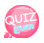# Array

Until now, we have been putting one value into
one variable, but there are many cases where a large amount of variables must be contained in one variable.
In this case, we put it in an array.
When importing any data from the database, it is often used in an array.

In other words, arrays can hold multiple values.
\$A = "my house"; I declared it like this. Using arrays, you can put your home in \$a, and you can put information like "robot", "girlfriend", "company".
If you need to put the address as country, state, city, east, detail, etc. in the array
You can enter it as above.
If there is no array,
Should I enter it as above? Now let's talk about arrays.
To declare an empty array, set the value to array(); Enter. As follows

` \$myAddress = array();`

You can also enter the value immediately.

``` \$userAddress = array('japan','tokyo','shibuyaku','1293-21');
```

Print an array.
Let's print out the values ​​of \$userAddress.
If you look at the array values, there are japan, tokyo, shibuyaku, and 1293-21. These values ​​have their own address in the array.
Start with 0 from the left. In order to print japan, the address starts at 0 from the left, so if you write the following output statement, japan will be printed.

```<?php
echo \$userAddress; //Open [in array variable, write address 0 and close again ]
?>```

how is it? Is it simple? So if you want to print a shibuyaku other than japan, the number is 2, because it starts at 0.

``` <?php
?>```

You can also print out the whole using the for statement.
Because address is a number, you can put a variable in the address. Let's try it
There are four values ​​in the array. To find the number of arrays, use the count() function. Very simple.
This way, there are 4 values, so it prints 4.

```<?php
?>```

So let's print it using the for statement.

```<?php

for(\$i = 0; \$i <= \$userAddressCount; \$i++){
}
?>```

In the source above, count(\$userAddress)-1; Does -1 at.
The reason is that the length of the array is 4, so we use -1 to use it in the for statement.
This is because address numbers start from 0, so 4, address numbers such as 0, 1, 2, and 3 are output.

And if you use \$userAddress [\$i] in the for statement, \$i is set to 0 in the for statement and moved up to 3 by the condition, so when you print
is due to be carried out.
In other words, since the array number is 4, the array address is 4, and since the array address starts from 0, if -1 is not used, the for statement executes 0, 1, 2, 3, 4 5 times from 0 to 4, so -1 is 0 To run 1,2,3.
The reason why I used -1 in the for statement so far was to print the contents of the array in an uncomfortable way to explain foreach.
If you follow the above source, it's annoying because you have to print the number while thinking about it

So there's also a way to print it at once, which is foreach? Using this is really simple.

```<?php
echo \$ua.'';
}
?>```

how is it? Is it simple? foreach(declare a variable to be used in an array as output statement) This is the end. ^^;

Index is automatically appended to the array, starting with 0 as a number. Since this is represented as a number, it can be difficult depending on what the 0 means. You can specify this address number yourself. Then shall we do it together? When declaring an array, \$userAddress = array('japan', 'tokyo', 'shibuyaku', '1293-21'); Don't declare it like this. Thus \$userAddress = array( 'nation' => 'japan', 'do' => 'tokyo', 'ku' => 'shibuyaku', 'bunji' => '1293-21'); And when printing, write the address name instead of numbers. Like this \$userAddress ['nation']; how is it? Easy?

```<?php
```<?php